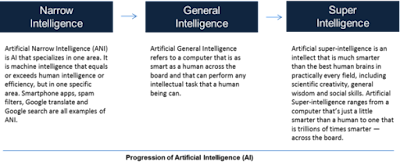## Cryptography after the Quantum Era (PQC).

### In the last decade, significant developments in quantum computing have reassured the scientific community of the need to develop quantum-resistant cryptosystems.

• Quantum computers represent a danger to conventional public-key encryption based on number theory, thus Post-Quantum Cryptography (PQC) has emerged as the preferable alternative (i.e., integer factorization or discrete logarithms).

Cryptosystems that are safe against assaults launched on classical computers and possibly quantum computers may be designed using:

1. lattice-based cryptography,
2. multivariate cryptography,
3. hash-based cryptography schemes,
4. isogeny-based cryptography,
5. and code-based encryption.

• As a result, these methods are known as PQC (Post Quantum Cryptography) algorithms.

### Cryptography methods based on lattices are easy to build and provide a solid demonstration of security.

• The shortest vector problem (SVP), which involves estimating the minimum Euclidean length of a lattice vector for any basis, is the foundation of lattice-based encryption.
• The worst-case quantum polynomial time to solve SVP is approximately exp(O(√ n)).
• SVP's complexity is polynomial in n even with the processing capability of a quantum computer.
• One of the numerous issues in the lattice family is Short Integer Solutions (SIS).
• If the SVP is difficult in the worst situation, SIS issues are secure in the average scenario.

### The fundamental assumptions of code-based cryptography systems are that the generator matrix and random matrix are indistinguishable and that generic decoding is difficult.

• Because they are based on a well-studied issue, these methods take a conservative approach to public key encryption/key encapsulation.
• If the key size is decreased, this class of algorithms becomes susceptible.
• Researchers have proposed methods for reducing key size without jeopardizing security.
• The complexity of solving the finite field multivariate polynomial (MVP) problem inspires multivariate cryptography.

### MVP issues are NP-hard to solve.

• MVPs are NP-complete problems if all equations are quadratic over GF.
• Despite the fact that certain MVP-based methods have been proven to be weak, the PQC signature technique provides for competitive signature sizes.
• The security characteristics of the underlying symmetric primitives, particularly cryptographic hash functions, are used to create hash-based digital signatures (leveraging properties of collision resistance and second pre-image resistance).

The National Institute of Standards and Technology (NIST) stated in that it will launch a standardization project to establish quantum-resistant standards for Key Encapsulation Mechanism (KEM) and Public Key Encryption (PKE), as well as digital signatures.

## NIST specified five distinct security strengths directly linked to NIST standards in symmetric cryptography in the request for proposals: Security Level :

1. Algorithm is at least as difficult to crack as AES (but it is less quantum resistant—Exhaustive Key Search).
2. Algorithm is at least as difficult to crack as SHA (strong in terms of quantum resistance—Collision Search).
3. Algorithm is at least as difficult to crack as AES (and is stronger in terms of quantum resistance—Exhaustive Key Search).
4. Algorithm is at least as difficult to crack as SHA (very strong quantum resistance—Collision Search).
5. Algorithm is at least as difficult to crack as AES (the strongest in terms of quantum resistance—Exhaustive Key Search).

### The NIST PQC Competition's first round began in December and received entries, from which digital signature contenders and KEM/PKE methods were selected.

• The NIST PQC Competition's second round candidates were revealed in January: digital signature candidates and KEM/PQC schemes.
• Just as the current work is going to print, NIST has officially announced a third cycle, which will begin in June.

###~ Jai Krishna Ponnappan